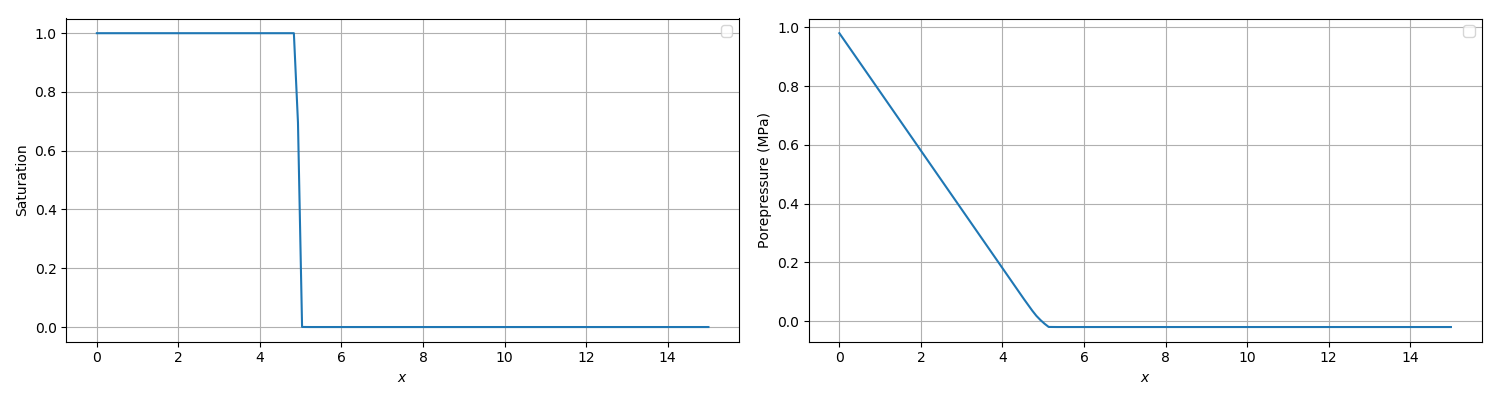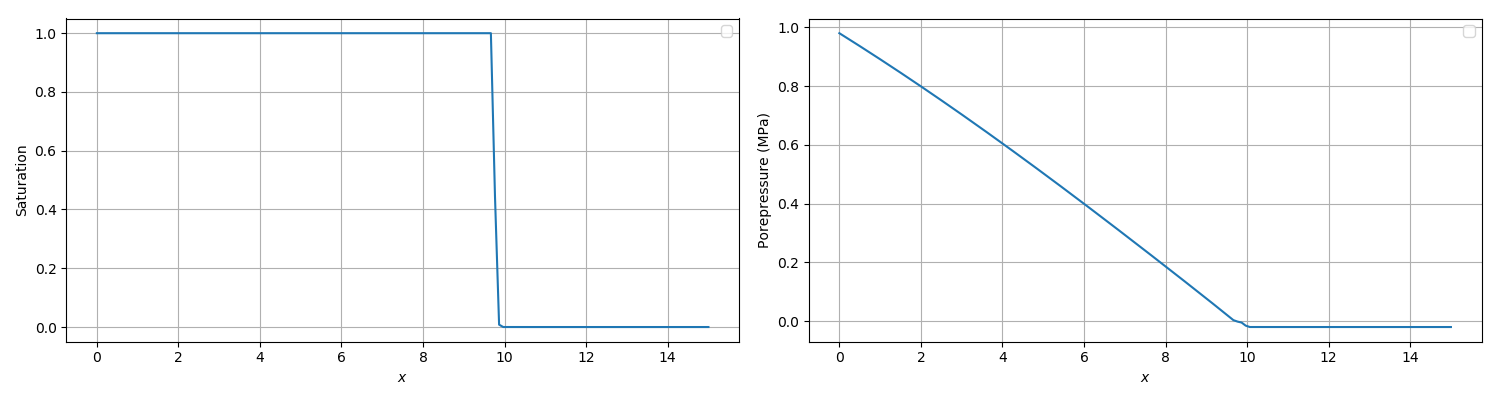# Injection ala Buckley and Leverett

PorousFlow is compared with a simple single-phase one-dimensional Buckley-Leverett problem (Buckley and Leverett, 1942). The single-phase fluid moves in a region m. A fully-saturated front initially sits at position , while the region is initially unsaturated. With zero suction function , there is no diffusion of the sharp front, and it progresses towards the right (increasing ).

# Buckley-Leverett 1-phase.
# The front starts at (around) x=5, and at t=50 it should
# have moved to x=9.6.  The version below has a nonzero
# suction function, and at t=50, the front sits between
# (about) x=9.6 and x=9.9.  Changing the van-Genuchten
# al parameter to 1E-4 softens the front so it sits between
# (about) x=9.7 and x=10.4, and the simulation runs much faster.
# With al=1E-2 and nx=600, the front sits between x=9.6 and x=9.8,
# but takes about 100 times longer to run.

[Mesh]
type = GeneratedMesh
dim = 1
nx = 150
xmin = 0
xmax = 15
[]

[GlobalParams]
PorousFlowDictator = dictator
compute_enthalpy = false
compute_internal_energy = false
[]

[Variables]
[./pp]
[./InitialCondition]
type = FunctionIC
function = 'max((1000000-x/5*1000000)-20000,-20000)'
[../]
[../]
[]

[Kernels]
[./mass0]
type = PorousFlowMassTimeDerivative
fluid_component = 0
variable = pp
[../]
[./flux0]
type = PorousFlowAdvectiveFlux
fluid_component = 0
variable = pp
gravity = '0 0 0'
[../]
[]

[BCs]
[./left]
type = DirichletBC
variable = pp
boundary = left
value = 980000
[../]
[]

[AuxVariables]
[./sat]
family = MONOMIAL
order = CONSTANT
[../]
[]

[AuxKernels]
[./sat]
type = MaterialStdVectorAux
variable = sat
execute_on = timestep_end
index = 0
property = PorousFlow_saturation_qp
[../]
[]

[UserObjects]
[./dictator]
type = PorousFlowDictator
porous_flow_vars = 'pp'
number_fluid_phases = 1
number_fluid_components = 1
[../]
[./pc]
type = PorousFlowCapillaryPressureVG
m = 0.8
alpha = 1e-3
[../]
[]

[Modules]
[./FluidProperties]
[./simple_fluid]
type = SimpleFluidProperties
bulk_modulus = 2e6
viscosity = 1e-3
density0 = 1000
thermal_expansion = 0
[../]
[../]
[]

[Materials]
[./temperature]
type = PorousFlowTemperature
[../]
[./ppss]
type = PorousFlow1PhaseP
porepressure = pp
capillary_pressure = pc
[../]
[./massfrac]
type = PorousFlowMassFraction
[../]
[./simple_fluid]
type = PorousFlowSingleComponentFluid
fp = simple_fluid
phase = 0
[../]
[./permeability]
type = PorousFlowPermeabilityConst
permeability = '1E-10 0 0  0 1E-10 0  0 0 1E-10'
[../]
[./relperm]
type = PorousFlowRelativePermeabilityCorey
n = 2
phase = 0
[../]
[./porosity]
type = PorousFlowPorosityConst
porosity = 0.15
[../]
[]

[Preconditioning]
active = andy
[./andy]
type = SMP
full = true
petsc_options_iname = '-ksp_type -pc_type -snes_atol -snes_rtol -snes_max_it'
petsc_options_value = 'gmres bjacobi 1E-10 1E-10 20'
[../]
[]

[Functions]
[./timestepper]
type = PiecewiseLinear
x = '0    0.01 0.1 1   1.5 2   20  30  40  50'
y = '0.01 0.1  0.2 0.3 0.1 0.3 0.3 0.4 0.4 0.5'
[../]
[]

[Executioner]
type = Transient
end_time = 50
[./TimeStepper]
type = FunctionDT
function = timestepper
[../]
[]

[VectorPostprocessors]
[./pp]
type = LineValueSampler
start_point = '0 0 0'
end_point = '15 0 0'
num_points = 150
sort_by = x
variable = pp
[../]
[./sat]
type = LineValueSampler
start_point = '0 0 0'
end_point = '15 0 0'
num_points = 150
sort_by = x
variable = sat
[../]
[]

[Outputs]
file_base = bl01
[./csv]
type = CSV
sync_only = true
sync_times = '0.01 50'
[../]
[./exodus]
type = Exodus
execute_on = 'initial final'
[../]
[]

(modules/porous_flow/test/tests/buckley_leverett/bl01.i)

This is a difficult problem to simulate numerically as maintaining the sharp front is hard. The front's speed is independent of the relative permeability, since the fluid is flowing from a fully-saturated region (where ). This problem is therefore a good test of the full upwinding.

In the simulation listed above, the pressure at the left boundary is kept fixed at MPa, while the right boundary is kept fixed at Pa, so the difference is MPa. The medium's permeability is set to and its porosity is . It is not possible to use a zero suction function in the MOOSE implementation, but using the van Genuchten parameters Pa and approximates it. The fluid viscosity is Pa.s.

The initial condition is (1) which is shown in Figure 1. With the suction function defined above this gives (2)Figure 1: Initial setup of the Buckley-Leverett problem where porepressure is a piecewise linear function. The region on the left is fully saturated, while the region on the right has saturation 0.000006. During simulation the value of porepressure on the left boundary is held fixed.

Good approximations for the pressure and the front position may be determined from (3) which has solution (4) For the parameters listed above, the front will be at position m at s. This solution is only valid for zero capillary suction. A nonzero suction function will tend to diffuse the sharp front.

With coarse meshes it is impossible to simulate a sharp front, of course, since the front is spread over at least one element.

Figure 2 shows the results from a MOOSE simulation with a uniform mesh of size m. At s the front in this simulation sits at m as desired. However, the simulation takes seconds to complete due to the very low values of saturation obtained for van Genuchten Pa. Other simulations give similar results but run much faster. For instance, the test suite listed above uses the van-Genuchten parameter Pa, but the front diffuses a little into the unsaturated region (the front sits between m and m at s).Figure 2: The MOOSE solution of the Buckley-Leverett problem at time 50s. Left: saturation. Right: porepressure. The front sits at x 9.6m as expected from the analytical solution.

1. E. Buckley and M. Leverett. Mechanism of fluid displacement in sands. Transactions of the AIME, 146:107–116, 04 1942. doi:10.2118/942107-G.[BibTeX]